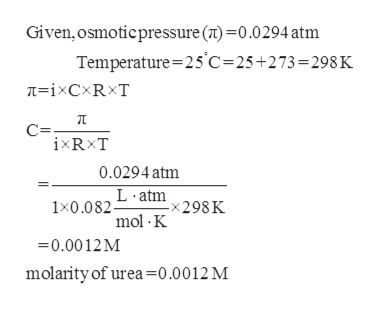Determine the molarity of each of the following solutions from its osmotic pressure at 25°C. Include the van't Hoff factor for the solution when the factor is given. II= 0.0294 atm for a solution of urea (CH4N2O):     M   II= 0.649 atm for a solution of KI, i = 1.90:

Question
Determine the molarity of each of the following solutions from its osmotic pressure at 25°C. Include the van't Hoff factor for the solution when the factor is given.

II= 0.0294 atm for a solution of urea (CH4N2O):     M
II= 0.649 atm for a solution of KI, i = 1.90:
Step 1

The molarity of the solution of ...help_outlineImage TranscriptioncloseGiven, osmoticpressure () 0.0294 atm Temperature 25 C-25+273=298 K C ixRxT 0.0294 atm 1x0.082Latm298K mol K 0.0012M molarity of urea=0.0012 M fullscreen

Want to see the full answer?

See Solution

Want to see this answer and more?

Our solutions are written by experts, many with advanced degrees, and available 24/7

See Solution
Tagged in

Physical Chemistry